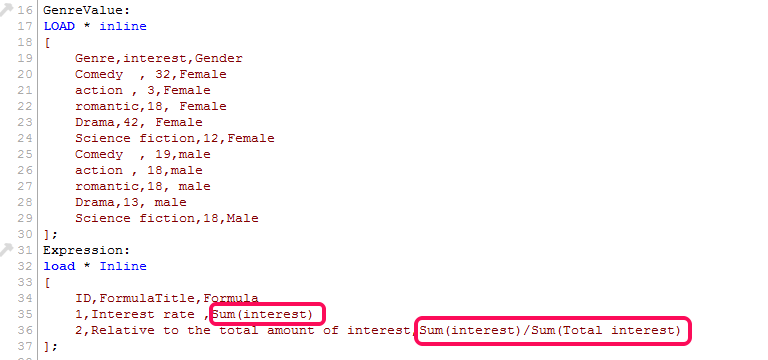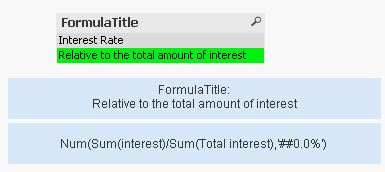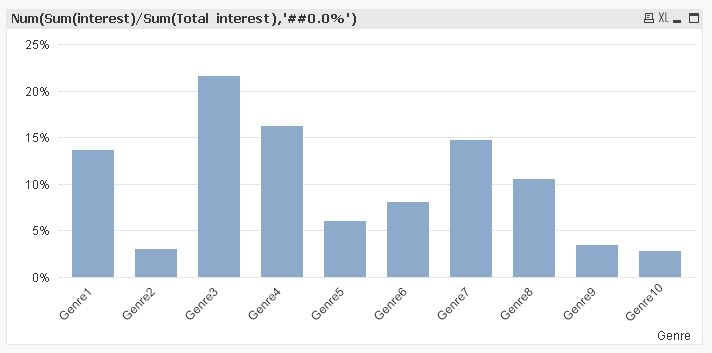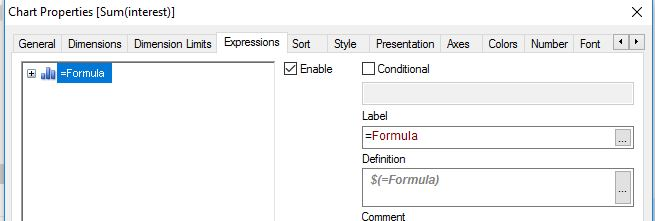# QlikView App Dev

Discussion Board for collaboration related to QlikView App Development.

Announcements
Skip the ticket, Chat with Qlik Support instead for instant assistance.
cancel
Showing results for
Did you mean:Anonymous
Not applicable

## How to Implement Dynamically Expression From Load Inline

Hello Dear

i want Implement chart Dynamically Expression   From Script

In the event that user select formula-title list Box ,show Related expression from formula-title and set on chart1 Solution

Accepted SolutionsMVP

Hi,

maybe one solution could be:```GenreValue:
Ceil(Rand()*100) as interest,
'Gender'&Ceil(Rand()*2) as Gender
AutoGenerate 30;

Expression:
LOAD RecNo() as ID, * INLINE [
FormulaTitle, Formula
Interest Rate, Sum(interest)
Relative to the total amount of interest, "Num(Sum(interest)/Sum(Total interest),'##0.0%')"
];
```

The Magic of Variables

The Magic of Dollar Expansions

The Little Equals Sign

hope this helps

regards

MarcoMVP

Hi,

maybe one solution could be:```GenreValue:
Ceil(Rand()*100) as interest,
'Gender'&Ceil(Rand()*2) as Gender
AutoGenerate 30;

Expression:
LOAD RecNo() as ID, * INLINE [
FormulaTitle, Formula
Interest Rate, Sum(interest)
Relative to the total amount of interest, "Num(Sum(interest)/Sum(Total interest),'##0.0%')"
];
```

The Magic of Variables

The Magic of Dollar Expansions

The Little Equals Sign

hope this helps

regards

MarcoCommunity Browser